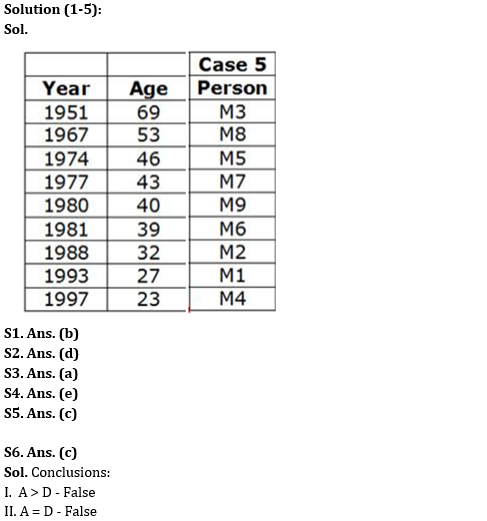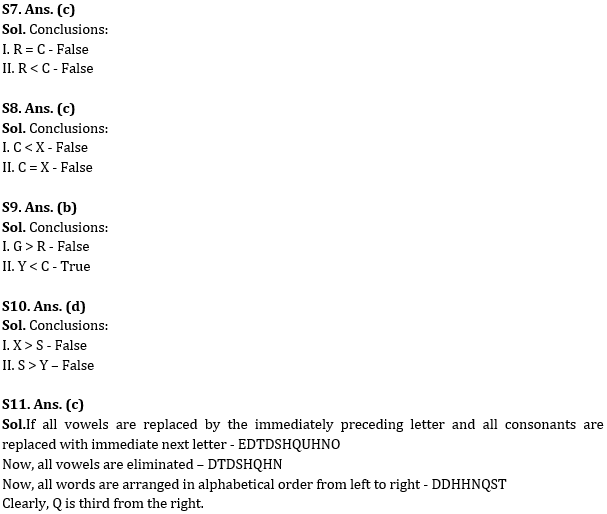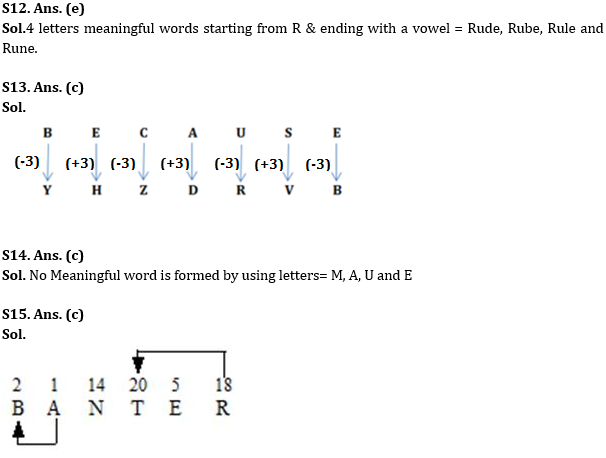Latest Banking jobs   »

# Reasoning Ability Quiz For RBI Grade B/ ECGC PO/ SIDBI Grade A Prelims 2022- 18th May

Directions (1-5): Read the following information carefully to answer the questions based on it.
Nine persons M1, M2, M3, M4, M5, M6, M7, M8 and M9 were born in different years viz., 1988, 1981, 1974, 1993, 1967, 1951, 1980, 1997 and 1977, not necessarily in the same order.
Note: Consider 2020 as the base year to calculate their age.
M8 is older than M9. M1 is younger than M5. M2 is either 23 years old or 32 years old. M5 is older than M2 but younger than M3. There are as many persons born after M4 as before M3. Only one person was born between M6 and M7. M6 is younger than M9. Four persons were born between M1 and M5.

Q1. What is the sum of the ages of M6 and M7?
(a) 86
(b) 82
(c) 115
(d) 66
(e) None of these

Q2. Who among the following is 39 years old?
(a) M7
(b) M8
(c) M9
(d) M6
(e) None of these

Q3. Who among the following is the oldest one?
(a) M3
(b) M8
(c) M7
(d) M9
(e) None of these

Q4. How many persons were born after M9?
(a) 3
(b) 8
(c) 7
(d) 5
(e) None of these

Q5. What is the sum of the ages of M3 and M8?
(a) 92
(b) 115
(c) 122
(d) 99
(e) None of these

Directions (6-10): In the following questions assuming the given statement to be true, find which of the conclusion(s) among given conclusions is/are definitely true and then give your answers accordingly.

Q6. Statements: C ≥ D < E ≥ F, A = B ≥ C, F > G < H
Conclusions:
I. A > D
II. A = D
(a) Only I is true
(b) Only II is true
(c) Either I or II is true
(d) Neither I nor II is true
(e) Both I and II are true

Q7. Statements: X ≤ C ≥ S > D, R ≤ T = X, Q = E ≥ R
Conclusions:
I. R = C
II. R < C
(a) Only I is true
(b) Only II is true
(c) Either I or II is true
(d) Neither I nor II is true
(e) Both I and II are true

Q8. Statements: R ≥ C ≤ V, T ≥ S = R, V = E ≤ Y = X
Conclusions:
I. C < X
II. C = X
(a) Only I is true
(b) Only II is true
(c) Either I or II is true
(d) Neither I nor II is true
(e) Both I and II are true

Q9. Statements: U ≤ R < T, G = H > Y ≤ U, T = C > V
Conclusions:
I. G > R
II. Y < C
(a) Only I is true
(b) Only II is true
(c) Either I or II is true
(d) Neither I nor II is true
(e) Both I and II are true

Q10. Statements: Z ≥ Y; X > T; Z ≥ S; Y ≥ X; R = Y
Conclusions:
I. X > S
II. S > Y
(a) Only I is true
(b) Only II is true
(c) Either I or II is true
(d) Neither I nor II is true
(e) Both I and II are true

Q11. Which of the following letter is third from the right if, all vowels are replaced by the immediately preceding letter and all consonants are replaced with immediate next letter in the word ‘DESCRIPTION’, and then all vowels are eliminated, after that all words are arranged in alphabetical order from left to right?
(a) S
(b) H
(c) Q
(d) N
(e) None of these

Q12. How many four-letter meaningful words (Starting with R and ending with a vowel) can be formed from the word BLUNDER without repeating any letters within that word?
(a) None
(b) One
(c) Two
(d) Three
(e) More than three

Q13. In a code language ‘PROVIDE’ is written as ‘MULYFGB’, then what will be code for ‘BECAUSE’ in the same language?
(a) YZHDRVB
(b) ZHYDRVB
(c) YHZDRVB
(d) ZYDHVBR
(e) None of these

Q14. Which letter is third from the left of the formed four-letter meaningful word by using the alphabets which are at first, second, fourth and eleventh place in the meaningful word ‘MANUFACTURE’, If more than one word is formed, then select ‘X’ as your answer? If no word is formed, select ‘Y’ as your answer.
(a) X
(b) M
(c) Y
(d) E
(e) U

Q15. How many such pairs of letters are there in the word BANTER, each of which has as many letters between them in the word (in both forward and backward directions) as they have between them in the English alphabetical series?
(a) None
(b) One
(c) Two
(d) Three
(e) More than three

Solutions#### Congratulations!